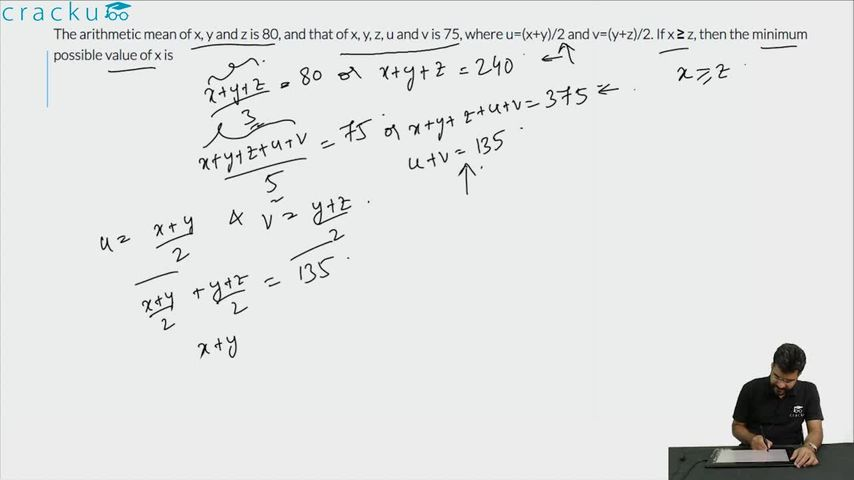Question 82

# The arithmetic mean of x, y and z is 80, and that of x, y, z, u and v is 75, where u=(x+y)/2 and v=(y+z)/2. If x ≥ z, then the minimum possible value of x is

Solution

Given that the arithmetic mean of x, y and z is 80.

$$\Rightarrow$$ $$\dfrac{x+y+z}{3} = 80$$

$$\Rightarrow$$ $$x+y+z = 240$$  ... (1)

Also,  $$\dfrac{x+y+z+v+u}{5} = 75$$

$$\Rightarrow$$ $$\dfrac{x+y+z+v+u}{5} = 75$$

$$\Rightarrow$$ $$x+y+z+v+u = 375$$

Substituting values from equation (1),

$$\Rightarrow$$ $$v+u = 135$$

It is given that u=(x+y)/2 and v=(y+z)/2.

$$\Rightarrow$$ $$(x+y)/2+(y+z)/2 = 135$$

$$\Rightarrow$$ $$x+2y+z = 270$$

$$\Rightarrow$$ $$y = 30$$   (Since $$x+y+z = 240$$)

Therefore, we can say that $$x+z = 240 - y = 210$$. We are also given that x ≥ z,

Hence, $$x_{min}$$ = 210/2 = 105.

### View Video Solution